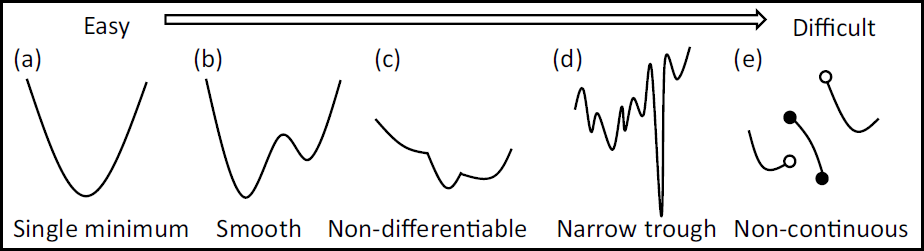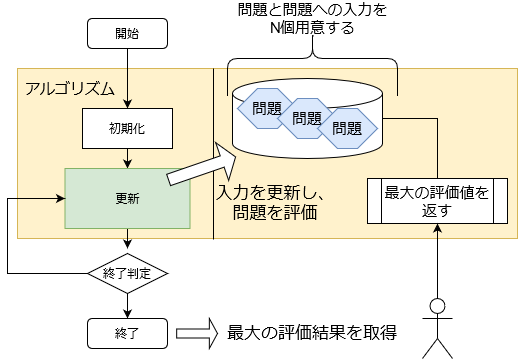# Introduction

We will mainly implement the metaheuristics algorithm in the optimization algorithm. Metaheuristics have the greatest advantage of being problem-independent, but It was difficult to understand how to approach the actual problem, so I summarized it.

What I want to do is

1. Generalize as clearly as possible to create a common interface to the problem
2. Compare each algorithm

is.

In addition, each algorithm will be published in a separate article and will be published little by little. (I will add a link when I post an article) The code can be found on github.

# Target algorithm

• If there is an algorithm you care about, we may add it.

# Target problem

The details of the problem are summarized in Problem. The implemented issues are:

# Comparison result

I compared the optimization algorithms (provisional) (to do)

The purpose of the combination optimization problem is to find the input (combination) to the function that gives the maximum (or minimum) result of the objective function when a certain objective function (problem) is given.(The figure is taken from Benchmark function summary for evaluating optimization algorithm)

One of the methods to solve a function like (e) is a metaheuristic algorithm.

## Generalization of the problem

The purpose of generalization is to express what kind of interface (input format or output format) should be prepared on the problem side when applying a problem to the algorithm introduced here. And the responsibility of the algorithm is to use only that interface to produce results. (Of course, the performance will improve if you understand the characteristics of each algorithm and tune it accordingly.)

In this article, the input and output of the problem side are defined as follows.The size of the input to the problem N
Input to the problem \vec{x}=(x_1,x_2,...,x_N)
One element of input to the problem(Input value) x_i (i=1...N)
1 element range Prob_{min}\leqq x_i \leqq Prob_{max}
The result of passing an array of input values ​​to the problem(Evaluation value) Score = Prob(\vec{x})

$Prob$ is an element that changes from problem to problem. The input is an n-dimensional array $(x_1, x_2, ..., x_N)$, where each $x$ has a range of values ​​specified by the problem side. When the problem side receives this array, it evaluates it and returns the evaluation value. It is the responsibility of the algorithm to maximize this evaluation value.

• Input values ​​are real values ​​for versatility. Therefore, depending on the problem, it may be necessary to make it a discrete value when evaluating.
• The maximum value is targeted. If you want to minimize it, the problem side will deduct it.

### Representing the interface in the program code

The class that represents the problem and the class that represents the input are separated.

• Problem class

The responsibility of the class that represents the problem is to create the input and evaluate it when it is received. Only the contents that must be implemented in common for each problem are described.

import random
class Problem():
def __init__(self, size):
self.size = size  #Input size to the objective function(N)

self.MIN_VAL = 0  #Minimum value of element(Designated for each problem)(Prob_min)
self.MAX_VAL = 1  #Maximum element value(Designated for each problem)(Prob_max)

def create(self, arr=None):
"""
Generates the input value.
If the argument is None, create a random input and
If a value is specified, that value will be used to create the input.
Problem Data will be described later
"""
o = ProblemData(self, self.size)
if arr is None:
arr = [ self.randomVal() for _ in self.size]
o.setArray(arr)
return o

def randomVal(self):
"""
A function that returns a random value for one element
"""
return self.MIN_VAL + random.random() * (self.MAX_VAL - self.domain.MIN_VAL)

def init(self):
"""
Problem initialization, optionally created
"""
raise NotImplementedError()

def eval(self, arr):
"""
Evaluate the input and return the result
This corresponds to the objective function part
"""
raise NotImplementedError()


• Input class for the problem

The responsibility of the input class is to operate the input and cache and return the evaluation value. Depending on the problem, it takes the longest time to output the evaluation value, so cache it on the input class side. This eliminates the need to think about the cache on the problem class side.

class ProblemData():
def __init__(self, domain, size):
self.domain = domain  #Problem class
self.size = size      #Input size to the objective function
self.arr = [ 0 for _ in range(self.size)]  #Input value
self.score = None     #Evaluation value(For cache)

def copy(self):
"""
Returns a copy of itself
"""
o = ProblemData(self.domain, self.size)
o.arr = [ x for x in self.arr]  # deepcopy
o.score = self.score
return o

def getArray(self):
"""
Returns the input value.
For safety, duplicate and return.
"""
return [ x for x in self.arr]

def setArray(self, arr):
"""
Set the input value.
"""
#Round with the lower and upper limits
for i in range(len(arr)):
if arr[i] < self.domain.MIN_VAL:
arr[i] = self.domain.MIN_VAL
if arr[i] > self.domain.MAX_VAL:
arr[i] = self.domain.MAX_VAL
self.arr = arr
self.score = None  #Clear cache

def getScore(self):
"""
Returns the evaluation value
"""
if self.score is not None:
return self.score
self.score = self.domain.eval(self.arr)
return self.score



## Generalization of algorithm

The algorithm has $M$ of problems. (You don't have to have it, but for now there are only algorithms with multiple problems)

By updating the input for each question, the goal is to find the question with the highest rating.

• In the implementation, it is assumed only when the evaluation value is maximized.

• Algorithm class

class IAlgorithm():

def init(self, problem):
"""
Initialization function
Associate the problem with the argument and also initialize the algorithm side
"""
raise NotImplementedError()

def step(self):
"""
One update on the algorithm side
"""
raise NotImplementedError()

def getMaxElement(self):
"""
Returns the problem of the maximum evaluation value in the algorithm
"""
raise NotImplementedError()

def getElements(self):
"""
Returns all problems used in the algorithm
"""
raise NotImplementedError()

def getScores(self):
"""
Returns the evaluation value of all problems used in the algorithm
"""
return [x.getScore() for x in self.getElements()]

def getMaxScore(self):
"""
Returns the maximum evaluation value in the algorithm
"""
return self.getMaxElement().getScore()



## main function

The above problem class and algorithm class are supposed to work as follows.

prob = Problem(N)  #Create a problem with N-dimensional input
alg = IAlgorithm()  #Specify algorithm

#Initialize
prob.init()